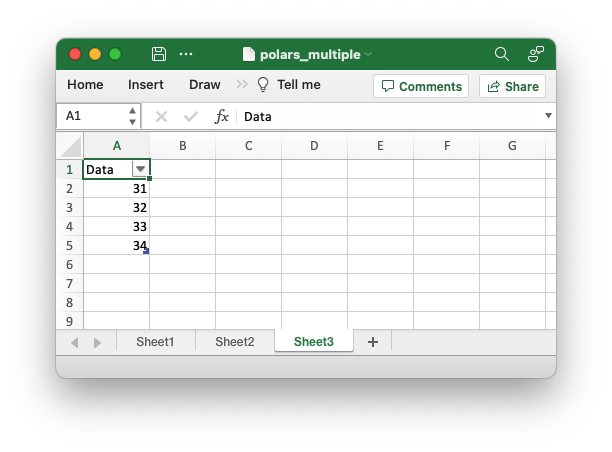# Example: Polars Excel with multiple dataframes

An example of writing multiple dataframes to worksheets using Polars and XlsxWriter. See Working with Polars and XlsxWriter for more details.```##############################################################################
#
# An example of writing multiple dataframes to worksheets using Polars and
# XlsxWriter.
#
# Copyright 2013-2023, John McNamara, jmcnamara@cpan.org
#

import xlsxwriter
import polars as pl

# Create some Polars dataframes from some data.
df1 = pl.DataFrame({"Data": [11, 12, 13, 14]})
df2 = pl.DataFrame({"Data": [21, 22, 23, 24]})
df3 = pl.DataFrame({"Data": [31, 32, 33, 34]})

with xlsxwriter.Workbook("polars_multiple.xlsx") as workbook:
df1.write_excel(workbook=workbook)
df2.write_excel(workbook=workbook)
df3.write_excel(workbook=workbook)
```